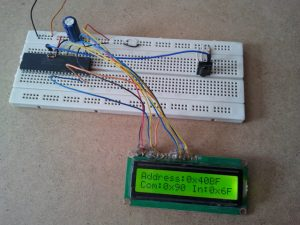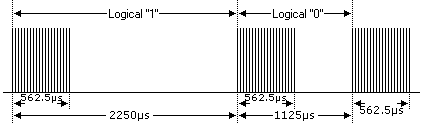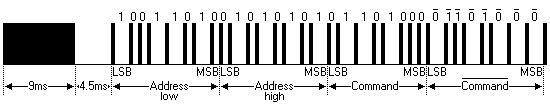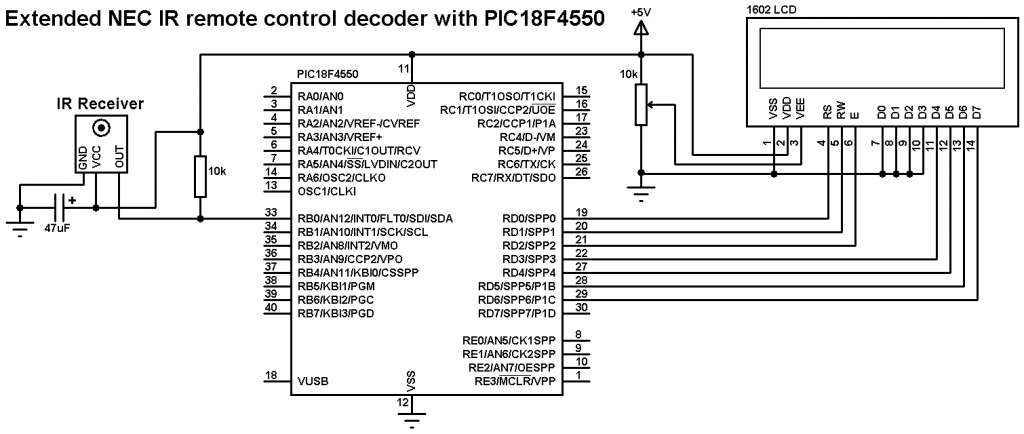# Extended NEC remote control decoder with PIC18F4550 – CCS C

Extended NEC protocol decoderThe NEC protocol uses pulse distance encoding of the bits. Each pulse is a 562.5µs long with carrier frequency of 38KHz. Logic bits are transmitted as follows:
Logic 0: 562.5µs pulse burst followed by a 562.5µs space, with a total transmit time of 1125µs     (562.5 x 2).
Logic 1: a 562.5µs pulse burst followed by a 1687.5µs (562.5 x 3) space, with a total transmit time of 2250µs (562.5 x 4).The complete extended NEC protocol message is started by 9ms burst followed by 4.5ms space which is then followed by the Address and Command. The address is 16-bit length and the command is transmitted twice (8 bits + 8 bits) where in the second time all bits are inverted and can be used for verification of the received message. The following drawing shows an extended NEC message example.NEC Protocol IR remote control decoder with PIC18F4550 microcontroller:
It is easy to decode IR remote control uses NEC protocol using microcontrollers.
Here Microchip PIC18F4550 microcontroller is used to decode IR remote controls which uses NEC and extended NEC protocol. Decoder circuit schematic is shown below.In this project PIC18F4550 internal oscillator is used @ 8MHz and MCLR pin function is disabled in the software.

NEC Protocol IR remote control decoder with PIC18F4550 CCS C code:
There are different ways to decode the NEC protocol for example using CCP module and Timer module, using Timer module or using port change interrupt…….. In this project I didn’t use any interrupt or Timer, I used delay command to make the code as simple as possible with time-out property and the code checks the IR signal with resolution of 50µs.
Programming hints:
From the decoder circuit schematic above the output of the IR receiver is connected to RB0 and when an IR signal is received RB0 pin goes from logic high (+5V) to logic low (0V).
The NEC message has 32 bits which are divided into address (16 bits), command (8 bits) and inverted command (8 bits).
The microcontroller waits until the IR receiver receives an IR signal which makes RB0 goes from logic high to logic low and the command used is:
while(input(PIN_B0));
After that a function named nec_remote_read() is called, this function checks if the received signal has NEC protocol form all the time.
The last function reads the 9ms burst using the following lines and if the pulse is more than 10ms (50µs x 200) or less than 8ms (50µs x 160) the function returns with false result which means the received signal doesn’t have NEC protocol form.
while((input(PIN_B0) == 0) && (count < 200)){
count++;
delay_us(50);}
if( (count > 199) || (count < 160))
return false;
The 4.5ms space is checked as the 9ms burst with small differences:
while((input(PIN_B0)) && (count < 100)){
count++;
delay_us(50);}
if( (count > 99) || (count < 60))
return false;
After that the microcontroller starts reading the 32 bits and keeps checking of NEC protocol form.
The following code is the complete CCS PIC C code written with compiler PCWHD version 5.051.

NEC Protocol IR remote control decoder with PIC18F4550 video:
The following video shows how this decoder works in a hardware circuit.

Reference:
http://www.sbprojects.com/

This site uses Akismet to reduce spam. Learn how your comment data is processed.